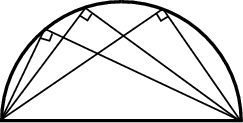Thales is credited with Thales' Theorem, which states that in a circle, the diameter always subtends a right angle from the circumference.

A generalisation of Thales' Theorem says that the angle subtended at the centre of a circle by a chord is exactly double the angle subtended at the circumference. Relatively easy to prove, it nonetheless forms the basis of a method of computing one's position from a collection of relative bearings.

CategoryMaths